Random Walks Random Walks on Graphs Random Walks

• Slides: 31Random Walks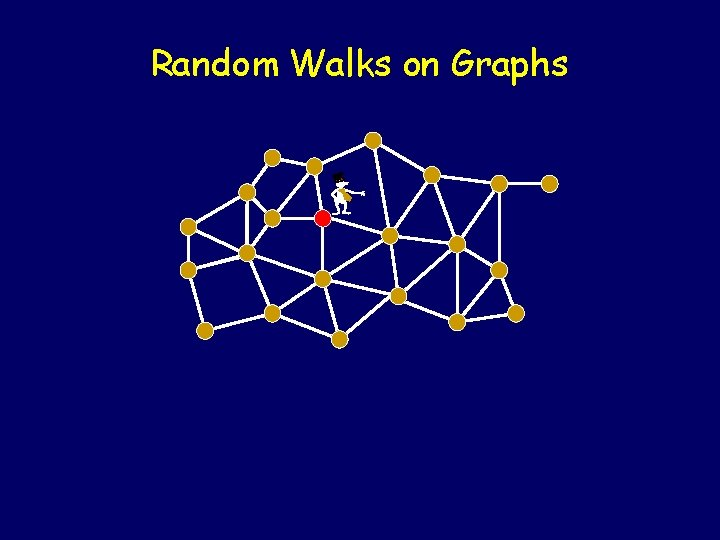Random Walks on Graphs -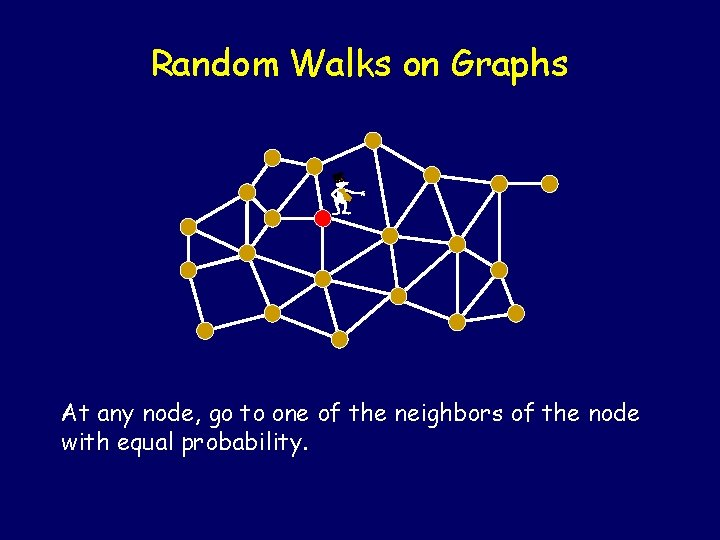Random Walks on Graphs - At any node, go to one of the neighbors of the node with equal probability.Random Walks on Graphs - At any node, go to one of the neighbors of the node with equal probability.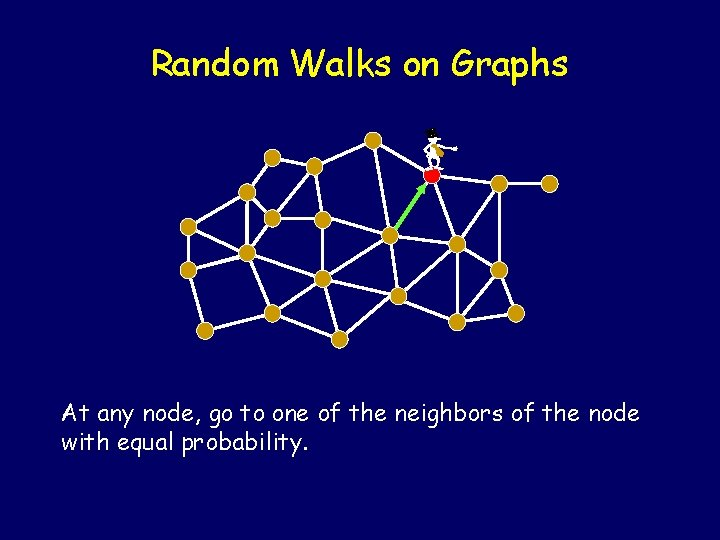Random Walks on Graphs - At any node, go to one of the neighbors of the node with equal probability.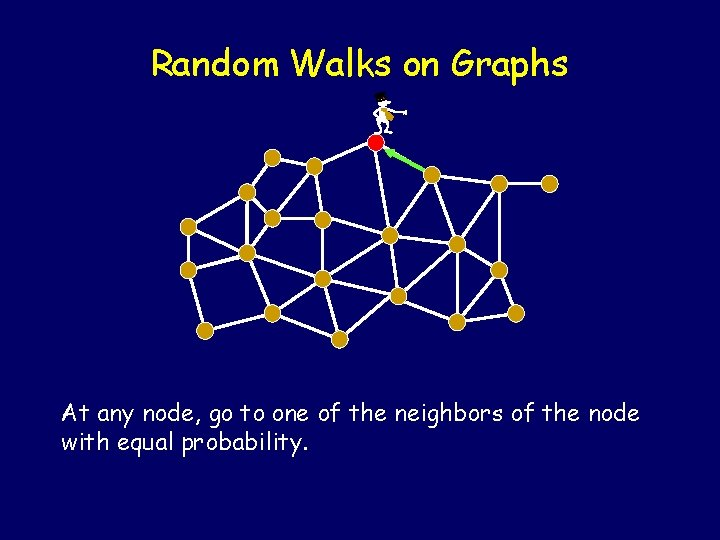Random Walks on Graphs - At any node, go to one of the neighbors of the node with equal probability.Random Walks on Graphs - At any node, go to one of the neighbors of the node with equal probability.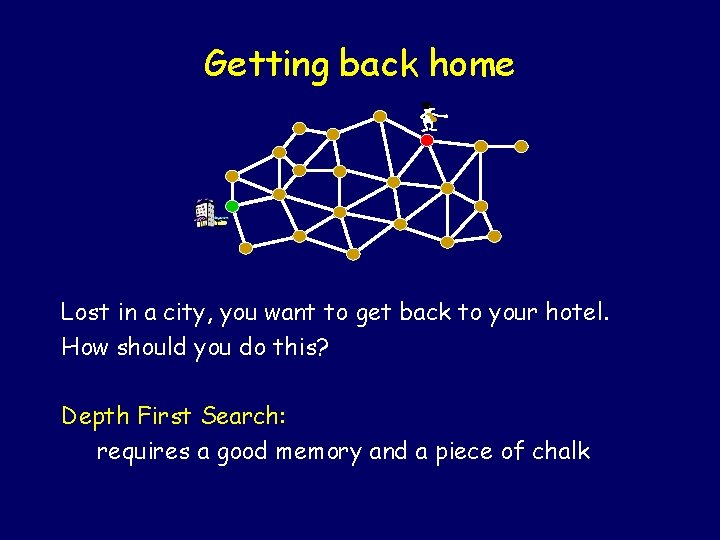Getting back home - Lost in a city, you want to get back to your hotel. How should you do this? Depth First Search: requires a good memory and a piece of chalk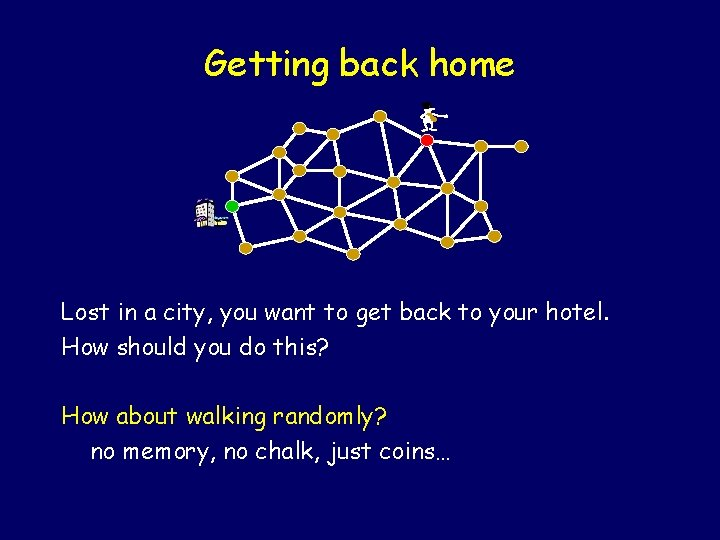Getting back home - Lost in a city, you want to get back to your hotel. How should you do this? How about walking randomly? no memory, no chalk, just coins…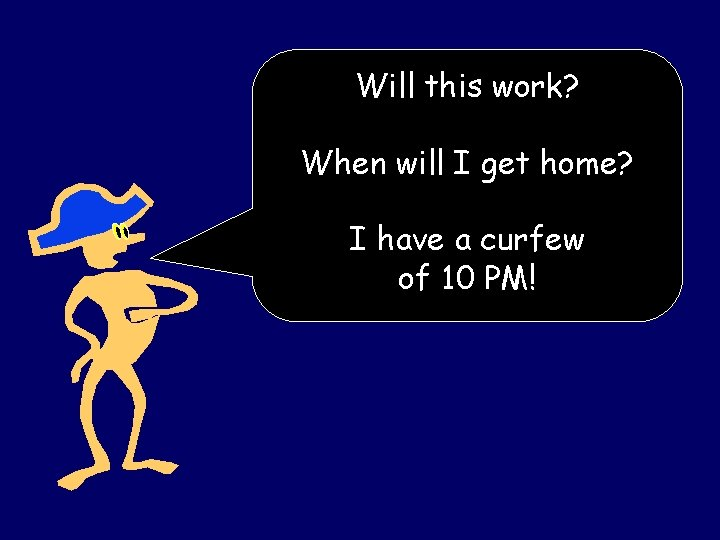Will this work? When will I get home? I have a curfew of 10 PM!Will this work? Is Pr[ reach home ] = 1? When will I get home? What is E[ time to reach home ]? I have a curfew of 10 PM!Relax, Dude! Yes, Pr[ will reach home ] = 1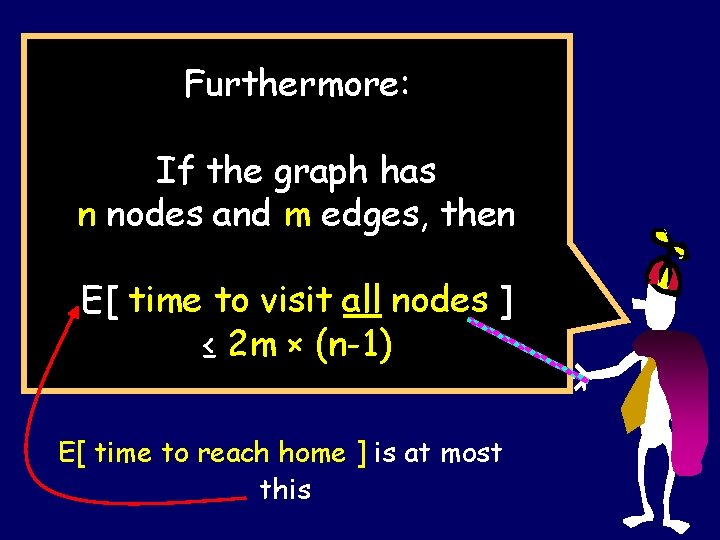Furthermore: If the graph has n nodes and m edges, then E[ time to visit all nodes ] ≤ 2 m × (n-1) E[ time to reach home ] is at most thisCover times Let us define a couple of useful things: Cover time (from u) Cu = E [ time to visit all vertices | start at u ] Cover time of the graph: C(G) = maxu { Cu }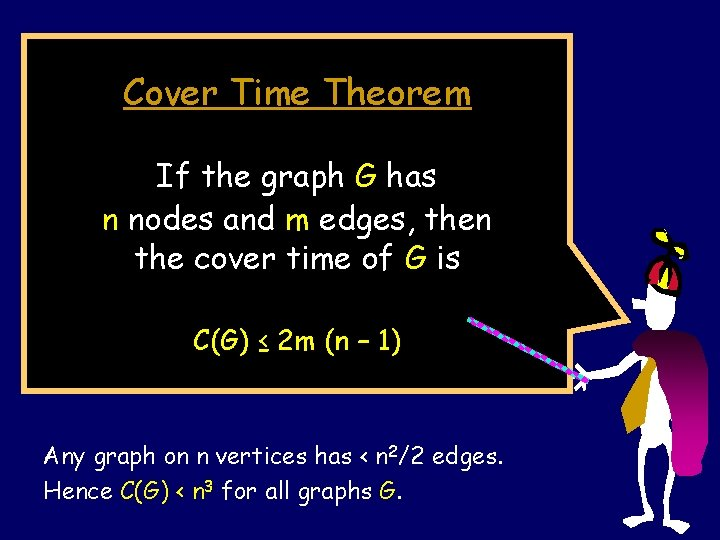Cover Time Theorem If the graph G has n nodes and m edges, then the cover time of G is C(G) ≤ 2 m (n – 1) Any graph on n vertices has < n 2/2 edges. Hence C(G) < n 3 for all graphs G.Let’s prove that Pr[ eventually get home ] = 1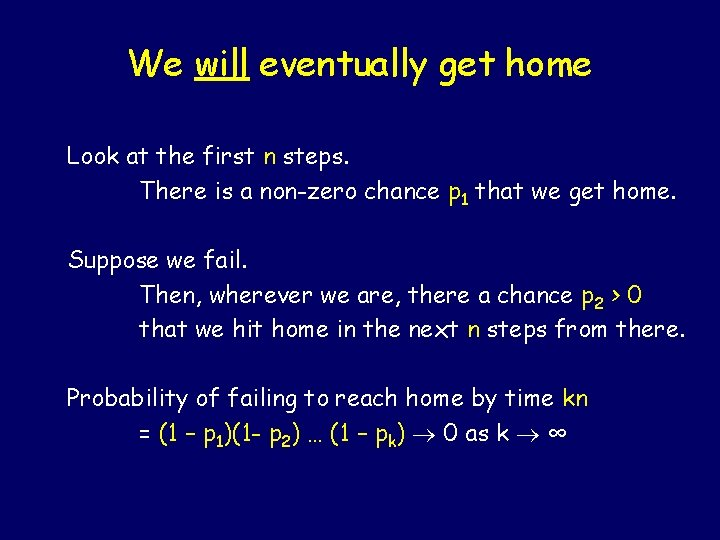We will eventually get home Look at the first n steps. There is a non-zero chance p 1 that we get home. Suppose we fail. Then, wherever we are, there a chance p 2 > 0 that we hit home in the next n steps from there. Probability of failing to reach home by time kn = (1 – p 1)(1 - p 2) … (1 – pk) 0 as k ∞In fact Pr[ we don’t get home by 2 k C(G) steps ] ≤ (½)k Recall: C(G) = cover time of G ≤ 2 m(n-1)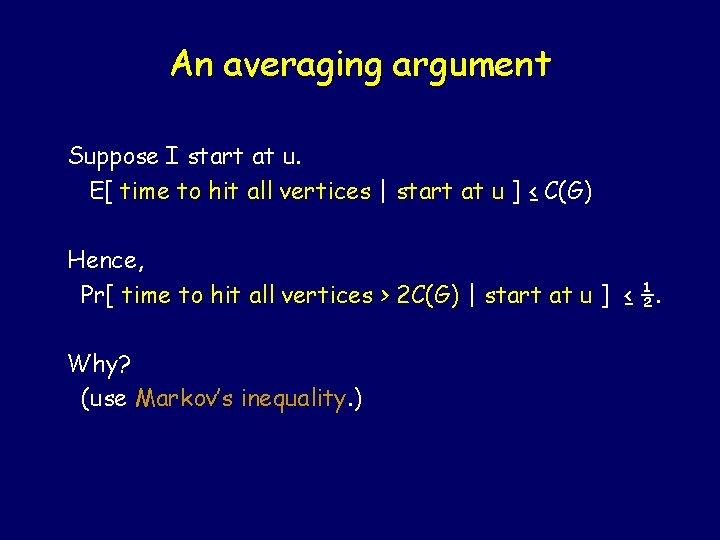An averaging argument Suppose I start at u. E[ time to hit all vertices | start at u ] ≤ C(G) Hence, Pr[ time to hit all vertices > 2 C(G) | start at u ] ≤ ½. Why? (use Markov’s inequality. )so let’s walk some more! Pr [ time to hit all vertices > 2 C(G) | start at u ] ≤ ½. Suppose at time 2 C(G), am at some node v, with more nodes still to visit. Pr [ haven’t hit all vertices in 2 C(G) more time | start at v ] ≤ ½. Chance that you failed both times ≤ ¼ !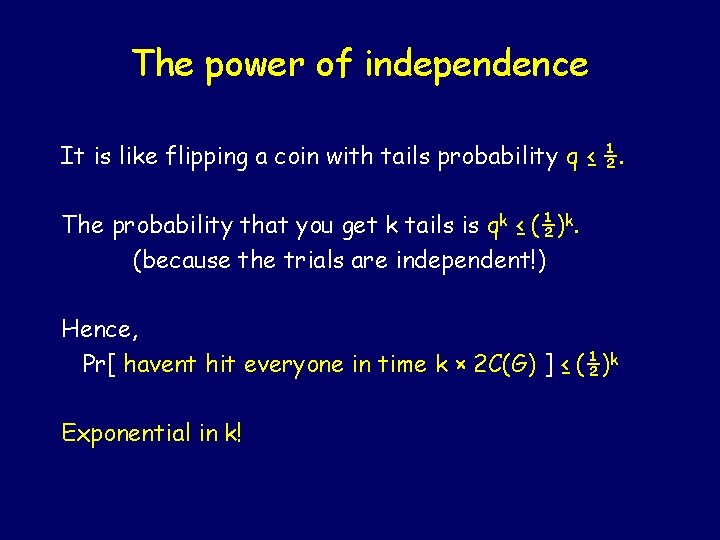The power of independence It is like flipping a coin with tails probability q ≤ ½. The probability that you get k tails is qk ≤ (½)k. (because the trials are independent!) Hence, Pr[ havent hit everyone in time k × 2 C(G) ] ≤ (½)k Exponential in k!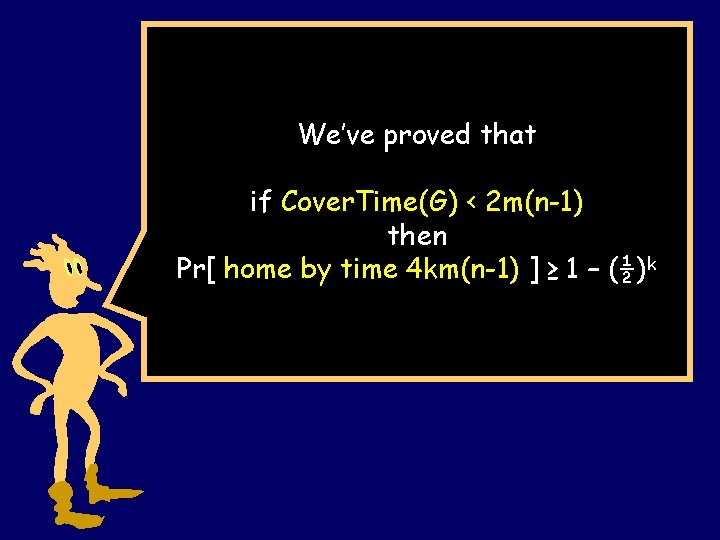We’ve proved that if Cover. Time(G) < 2 m(n-1) then Pr[ home by time 4 km(n-1) ] ≥ 1 – (½)kCover Time Theorem If the graph G has n nodes and m edges, then the cover time of G is C(G) ≤ 2 m (n – 1)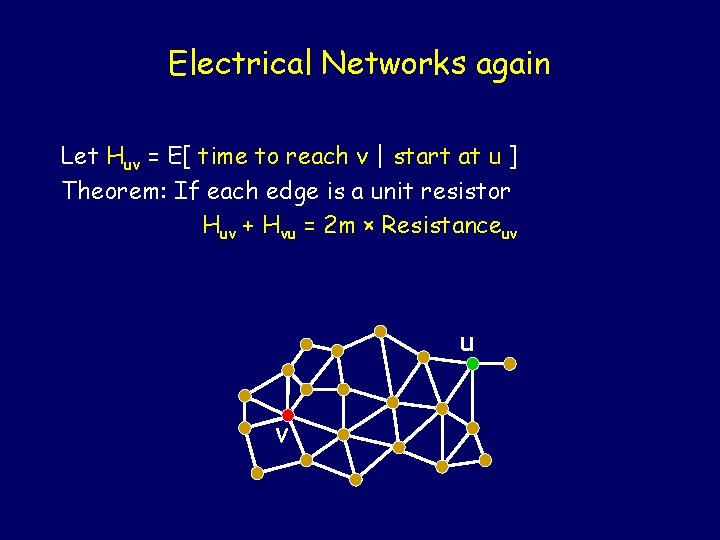Electrical Networks again Let Huv = E[ time to reach v | start at u ] Theorem: If each edge is a unit resistor Huv + Hvu = 2 m × Resistanceuv u v -Electrical Networks again Let Huv = E[ time to reach v | start at u ] Theorem: If each edge is a unit resistor Huv + Hvu = 2 m × Resistanceuv If u and v are neighbors Resistanceuv ≤ 1 Then Huv + Hvu ≤ 2 m u -v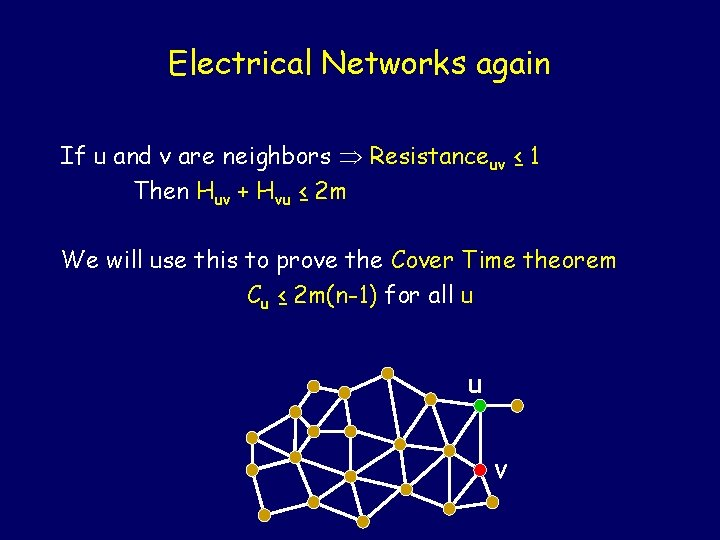Electrical Networks again If u and v are neighbors Resistanceuv ≤ 1 Then Huv + Hvu ≤ 2 m We will use this to prove the Cover Time theorem Cu ≤ 2 m(n-1) for all u u -vSuppose G is the graph 1 3 5 2 4 6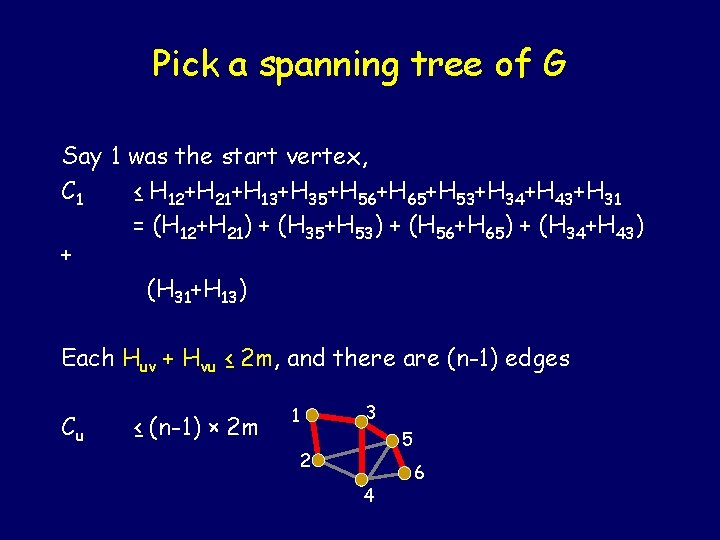Pick a spanning tree of G Say 1 was the start vertex, C 1 ≤ H 12+H 21+H 13+H 35+H 56+H 65+H 53+H 34+H 43+H 31 = (H 12+H 21) + (H 35+H 53) + (H 56+H 65) + (H 34+H 43) + (H 31+H 13) Each Huv + Hvu ≤ 2 m, and there are (n-1) edges Cu ≤ (n-1) × 2 m 1 3 2 4 -5 6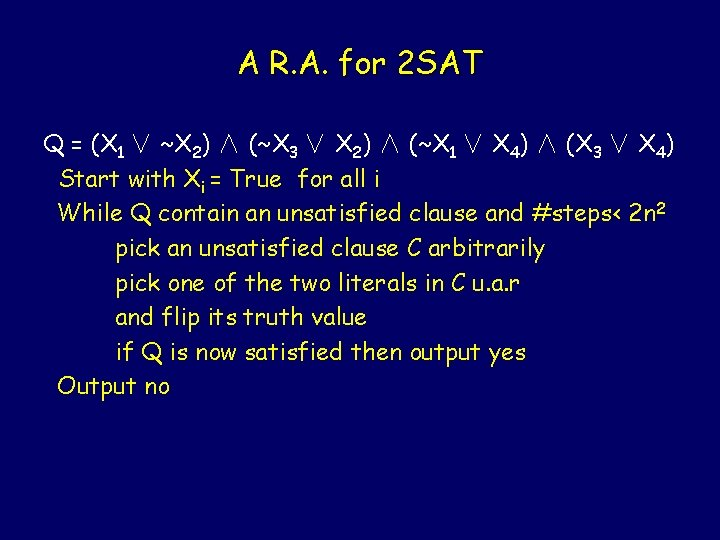A R. A. for 2 SAT Q = (X 1 ∨ ~X 2) ∧ (~X 3 ∨ X 2) ∧ (~X 1 ∨ X 4) ∧ (X 3 ∨ X 4) Start with Xi = True for all i While Q contain an unsatisfied clause and #steps< 2 n 2 pick an unsatisfied clause C arbitrarily pick one of the two literals in C u. a. r and flip its truth value if Q is now satisfied then output yes Output noAnaylsis 1. A* : any satisfying assignment 2. f(A): #variable of Q whose value in A is the same as A* 3. f(A) takes value in {0, 1, …, n} 4. if f(A) = n then A must be A* 5. at each step f(A) incr. or dec. by 1 6. at each step, Pr[f(A) incr. by 1] >= 1/2 0 n k